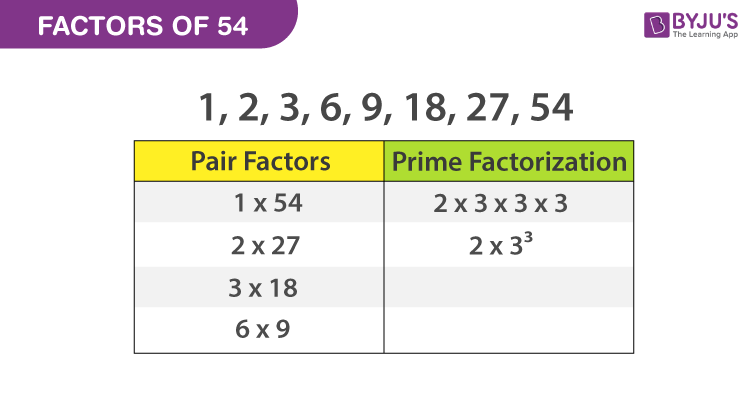# Factors of 54

The factors of 54 are the numbers, that can divide 54 completely or evenly. When a pair of factors are multiplied together to produce the 54, then they are said to be pair factors. The factors divide the number completely. Hence, these factors cannot be a fraction.

Factors of 54: 1,2,3,6,9,18,27 and 54

Factor pairs of the number 54 are the whole numbers which are not a fraction or decimal number. To find the factors of a number, 54, we will use the factorization method.Some important properties of factors are:

• A factor of any given number is its exact divisor
• For every number, 1 is a factor.
• The factor should be always less than or equal to the number itself
• The number itself is considered as the greatest factor of a number.

## What are the Factors of 54?

The factors of a number are the natural numbers that can divide the original number completely.

 Factors of 54 1, 2, 3, 6, 9, 18, 27, and 54

## How to Calculate Factors of 54?

To find factors of 54, we have to divide 54 by all natural numbers from 1 to 54.

• 54 ÷ 1 = 54
• 54 ÷ 2 = 27
• 54 ÷ 3 = 18
• 54 ÷ 6 = 9

## Prime Factors of 54 By Division Method

The number 54 is a composite and it should have prime factors. Now let us know how to calculate the prime factors of 54.

• The first step is to divide the number 54 with the smallest prime factor, say 2.

54 ÷ 2 = 27

• Again, when you divide 54 by 3, you will get a fractional number and it cannot be a   factor and continue with the next prime factor, say 3

27 ÷ 3 = 9

9 ÷ 3 = 3

3 ÷ 3 = 1

• Finally, we received the number 1 at the end of the division process. So that we cannot proceed further. So, the prime factors of 54 are written as 2 × 3 × 3 × 3 or 2x 33, where 2 and 3 are the prime numbers.

## Video Lesson on Prime Factors## Pair Factors of 54

To find the pair factors, multiply the two numbers in a pair to get the original number as 54, such numbers are as follows

1 × 54 = 54, (1, 54) is a pair factor of 54.

2 × 27 = 54, (2, 27) is a pair factor of 54

3 × 18 = 54, (3, 18) is a pair factor of 54

6 × 9 = 54, (6, 9) is a pair factor of 54

Therefore, the positive pair factors are (1, 54), (2, 27),(3, 18) and (6, 9).

## Solved Examples

Q.1: There are 54 bananas kept on a table. Mary has to distribute these bananas equally to her three children. How many bananas does each child will get?

Solution: Number of bananas = 54

Number of children = 3

Each child will get = 54/3 = 18 bananas.

Q.2: Write two times of all of the factors of 54.

Solution: Since we know the factors of the number 54 are 1, 2, 3, 6, 9, 18, 27, 54.

Thus, if we multiply 2 by each of the factors we get;

2,4,6,12,18,36,54 and 108

Q.3: What is the sum of all the factors of 54?

Solution: 1, 2, 3, 6, 9, 18, 27, 54 are the factors of 54

Now if we add all the factors, then;

1+2+3+6+9+18+27+54 = 120

Stay tuned with BYJU’S to know about factors of 54 and also learn the prime factors of other numbers. To clarify the doubts, watch interactive videos for better understanding.

## Frequently Asked Questions – FAQs

Q1

### How many factors are there for number 54?

The factors of 54 are 1, 2, 3, 6, 9, 18, 27 and 54. Hence, there are total 8 factors.
Q2

### Are the factors and multiples of 54 same?

Factors and multiples of 54 are different and not the same.
Q3

### What are the multiples of 54 in Maths?

The first ten multiples of 54 are 54, 108, 162, 216, 270, 324, 378, 432, 486 and 540.
Q4

### Which is the highest prime factor of 54?

Prime factorisation of 54 is 2 × 3 × 3 × 3. Therefore, the highest prime factor of 54 is 3.
Q5

### What are the positive pair factors of number 54?

The pair factors of whole number 54 are (1, 54), (2, 27), (3, 18), (6, 9).
 Links Related to Factors Factors of 50 Factor of 36 Factors of 48 Factors of 18 Factors of 24 Factors of 25 Factors of 42 Factors of 60 Factors of 35 Factors of 81 Factors of 75 Factors of 56
Test your knowledge on Factors of 54